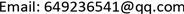1. 引言

2. 标准三季度HWT指数平滑模型

HWT 指数平滑模型由Brown, R.G.和Holt, C.C.首先提出，用于描述季节性时间序列的趋势   ，然后由J.W. Taylor进一步扩展到双季节性模式  。该模型良好的鲁棒性和预测的高精度使得其在季节时间预测方面得到了广泛的应用。由于有白天和夜晚、工作日和周末、夏天和冬天的区别，日模式、周模式和年模式的电力需求各有不同，周期属性在HWT指数平滑模型中通常被当做一个时间属性的模式参考。标准三种时间属性的HWT指数平滑模型可以描述为

L ( t ) = α [ d ( t ) − ( T ( t − 1 ) + S 1 ( t − s 1 ) + S 2 ( t − s 2 ) + S 3 ( t − s 3 ) ) ] + ( 1 − α ) ( L ( t − 1 ) + T ( t − 1 ) )

T ( t ) = β [ L ( t ) − ( L ( t − 1 ) + T ( t − 1 ) ) ] + ( 1 − β ) T ( t − 1 )

S 1 ( t ) = γ [ d ( t ) − ( L ( t − 1 ) + T ( t − 1 ) + S 2 ( t − s 2 ) + S 3 ( t − s 3 ) ) ] + ( 1 − γ ) S 1 ( t − s 1 )

S 2 ( t ) = λ [ d ( t ) − ( L ( t − 1 ) + T ( t − 1 ) + S 1 ( t − s 1 ) + S 3 ( t − s 3 ) ) ] + ( 1 − λ ) S 2 ( t − s 2 )

S 3 ( t ) = δ [ d ( t ) − ( L ( t − 1 ) + T ( t − 1 ) + S 1 ( t − s 1 ) + S 2 ( t − s 2 ) ) ] + ( 1 − δ ) S 3 ( t − s 3 )

d ^ ( t , k ) = L ( t ) + k T ( t ) + S 1 ( t − s 1 + k ) + S 2 ( t − s 2 + k ) + S 3 ( t − s 3 + k )

3. 四种季节HWT指数平滑模型

1) 每月底的电力消费量大于每月前十天的电力消费量；

2) 每月的最后一天的电力消费量最低。

L ( t ) = α [ d ( t ) − ( T ( t − 1 ) + S 1 ( t − s 1 ) + S 2 ( t − s 2 ) + S 3 ( t − s 3 ) + S 4 ( t − s 4 ) ) ] + ( 1 − α ) ( L ( t − 1 ) + T ( t − 1 ) )

T ( t ) = β [ L ( t ) − ( L ( t − 1 ) + T ( t − 1 ) ) ] + ( 1 − β ) T ( t − 1 ) ，

S 1 ( t ) = γ [ d ( t ) − ( L ( t − 1 ) + T ( t − 1 ) + S 2 ( t − s 2 ) + S 3 ( t − s 3 ) + S 4 ( t − s 4 ) ) ] + ( 1 − γ ) S 1 ( t − s 1 ) ，

S 2 ( t ) = λ [ d ( t ) − ( L ( t − 1 ) + T ( t − 1 ) + S 1 ( t − s 1 ) + S 3 ( t − s 3 ) + S 4 ( t − s 4 ) ) ] + ( 1 − λ ) S 2 ( t − s 2 ) ，

S 3 ( t ) = δ [ d ( t ) − ( L ( t − 1 ) + T ( t − 1 ) + S 1 ( t − s 1 ) + S 2 ( t − s 2 ) + S 4 ( t − s 4 ) ) ] + ( 1 − δ ) S 3 ( t − s 3 ) ，

S 4 ( t ) = ζ [ d ( t ) − ( L ( t − 1 ) + T ( t − 1 ) + S 1 ( t − s 1 ) + S 2 ( t − s 2 ) + S 3 ( t − s 3 ) ) ] + ( 1 − ζ ) S 4 ( t − s 4 ) ，

d ^ ( t , k ) = L ( t ) + k T ( t ) + S 1 ( t − s 1 + k ) + S 2 ( t − s 2 + k ) + S 3 ( t − s 3 + k ) + S 4 ( t − s 4 + k )

4. 应用四种时间周期属性方法进行电力预测4.1. 数据：新加坡的历史电力需求

4.2. 模型评价指标

A P E i = 1 n ∑ j = 1 N | d i j − d i j ^ d i j | ,     i = 1 , ⋯ , 48 ,   j = 1 , ⋯ , N (1)

M A P E i = 1 10 ∑ m = 1 10 | d i m − d i m ^ d i m | ,     i = 1 , ⋯ , 48 ,   m = 1 , ⋯ , 10 (2)

4.3. 仿真结果

5. 结论